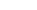•WE PROVIDEMULTIVARIATE
CALCULUS
HW HELP
We can help with
Matrix Operations
System of Equations
Vector Spaces
and much more
starts at \$25/hour.

# Multivariate Calculus Homework Help

## Calculus II and III Topics

• Differentiation
• Chain-Rule
• Implicit Differentiation

• Higher Derivatives and Extrema
• Higher Order Derivatives
• Taylor's Theorem
• Extrema: Maxima and Minima
• Second Derivative Rule, Hessian Matrix
• Lagrange Multipliers

• Vector Fields
• Divergence
• Curl

• Multiple Integrals
• Volumes
• Fubini's Theorem
• Double Integrals
• Triple Integrals
• Change of Variables: Polar, Cylindrical and Spherical

• Surface Integrals
• Parametrized Surfaces
• Normal Vectors
• Area of a Surface
• Flux Integrals

• Vector Analysis
• Green's Theorem
• Stokes' Theorem
• Gauss' Theorem

## Claim Your Free Solved Stats Problems!

* indicates required
Email Format

## Our Specialties

#### Differentiation

We can help with Differentiation, Partial Derivatives, Gradient, Chain-Rule and Implicit Differentiation. These topics appear commonly in Calculus II and III homework assignments.

#### Higher Derivatives and Extrema

The calculation of Higher Derivatives and Extrema requires of some consistent skill. Higher Order Derivatives, Taylor's Theorem, Maxima and Minima, Second Derivative Rule, Hessian Matrix and Lagrange Multipliers are topics that are usually considered as complicated by students. How experts can handle them for you.

#### Multiple Integrals

The concepts of Multiple Integrals, Volumes, Fubini's Theorem, Double Integrals, Triple Integrals and Change of Variables: Polar, Cylindrical and Spherical can absolutely be brain burners, that you may need help with. We can cover you!

#### System of Equations

Dealing with systems of equations can be tricky, as there are different methods that can be used and you sometimes are asked for one specific method. We can help YOU with all types of systems of equations.